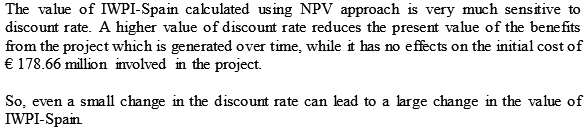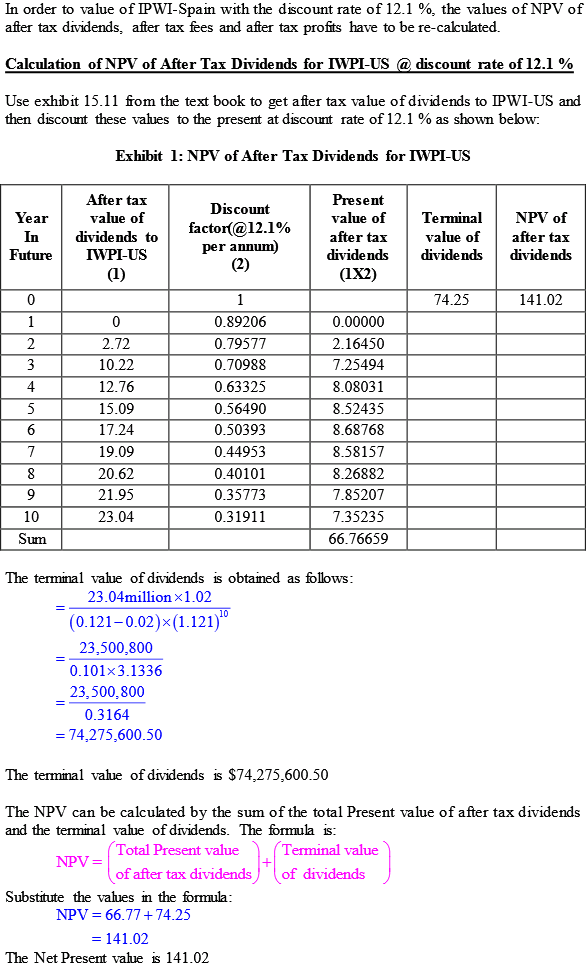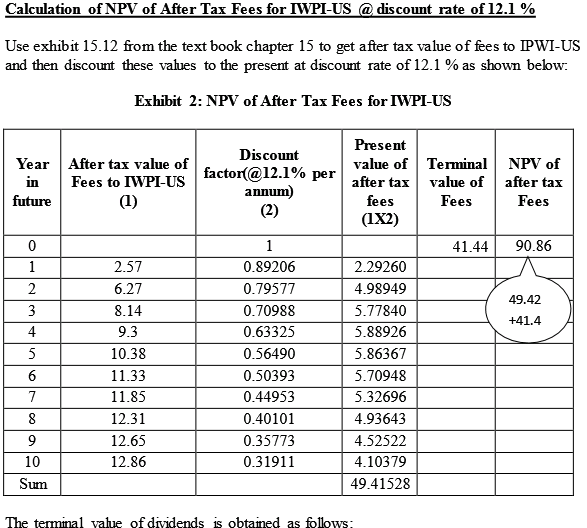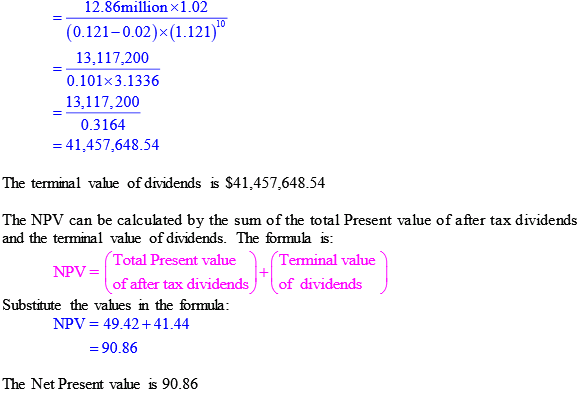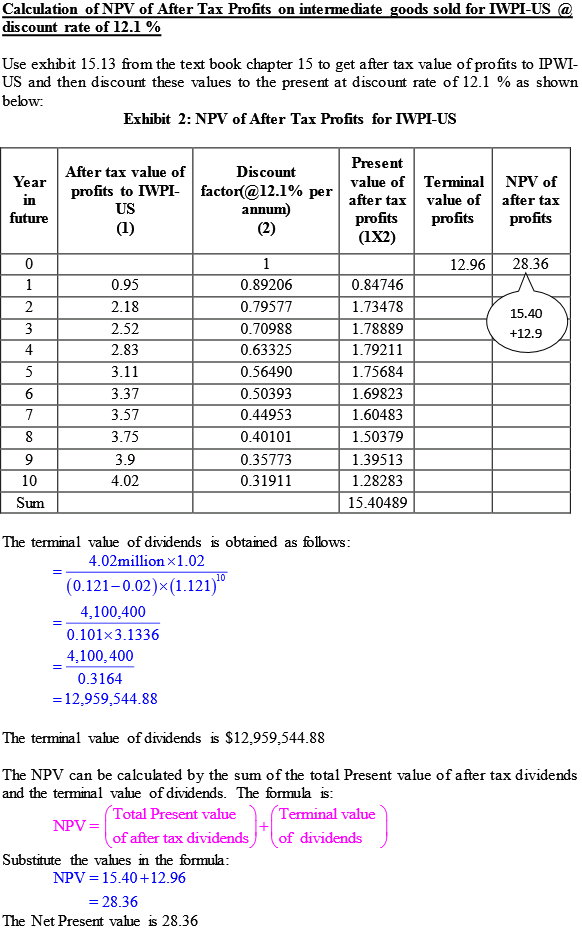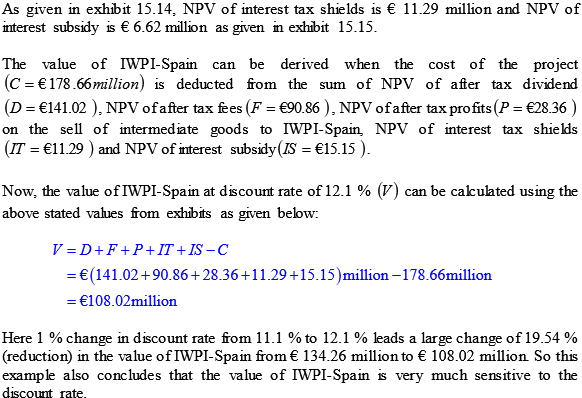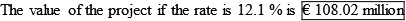# Quiz 15: International Capital Budgeting

The dividends that will occur more than 10 years in the future is the terminal dividend value representing the discounted present value of all expected future dividends in years 11 and beyond into the indefinite future. It can be calculated in two steps. Step 1: The terminal value of dividend in year 10 is taken as perpetuity that is growing at 2 %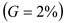with the long term euro inflation rate. The value of dividend in year 11 will be 2 % higher than the expected value of dividend in year 10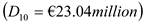. With the discount rate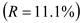the terminal value of dividend in year 10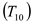is given by: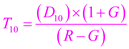Substitute the values in the formula: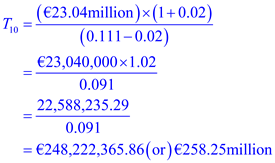The terminal value of dividend in year 10 is 258.25 million. Step 2: Now the terminal value in year 10is discounted to year 0 to get the present value of terminal value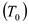as given below: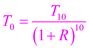Substitute the values in the formula: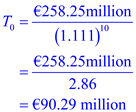The terminal value is 90.29 million. The adjusted net present value of IWPI-Spain is € 134.26 million. The dividends that will occur more than 10 years in the future; the terminal dividend is € 90.14 million. So the percentage of the adjusted net present value of IWPI-Spain project arises from the dividends that will occur more than 10 years in the future is: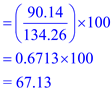The percentage of adjusted net present value is 67.13%